# Python 字典类型2

dict这个方法和列表的list方法一样，同样也可以把迭代对象，也就是可以被for循环的类型转换成字典类型，但前提是这个可迭代对象的每一个元素里都是存的两个值才可以。

``````l = [('name', '于谦'), ['age', 18]]
print(dict(l))``````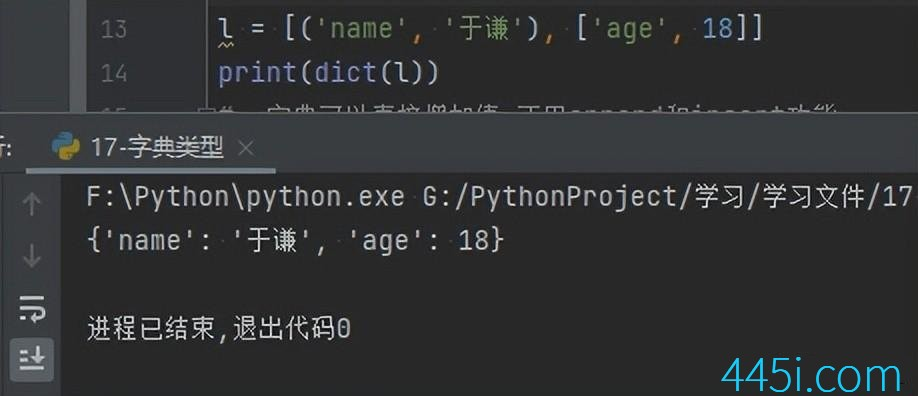``````l = [('name', '于谦'), ['age', 18], ['a', 'b', 'c']]
print(dict(l))``````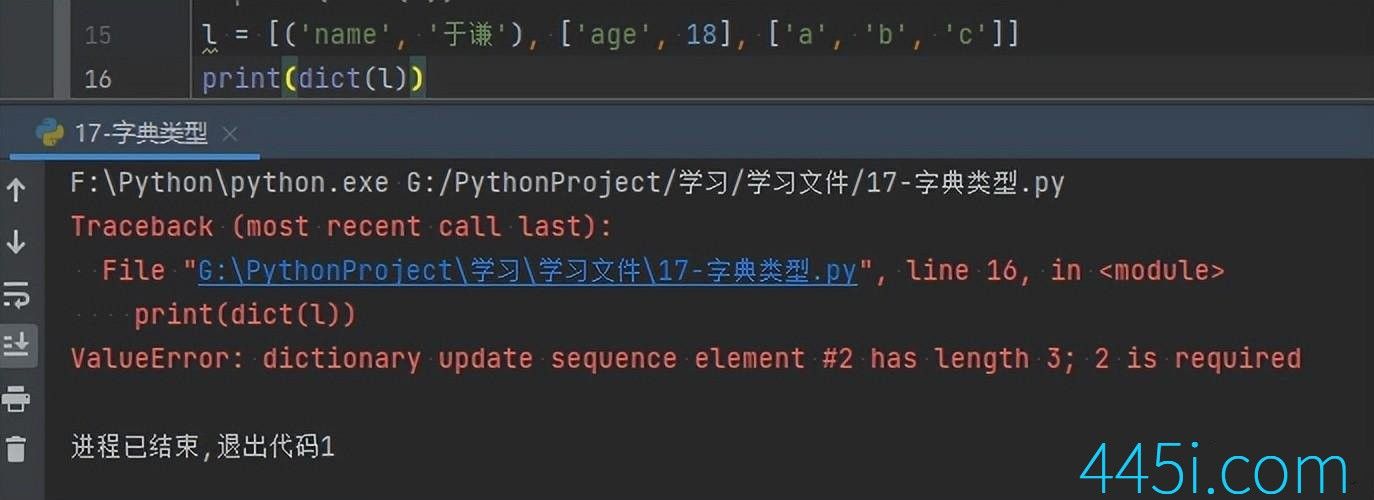``````l = [('name', '于谦'), ['age', 18], ['a']]
print(dict(l))``````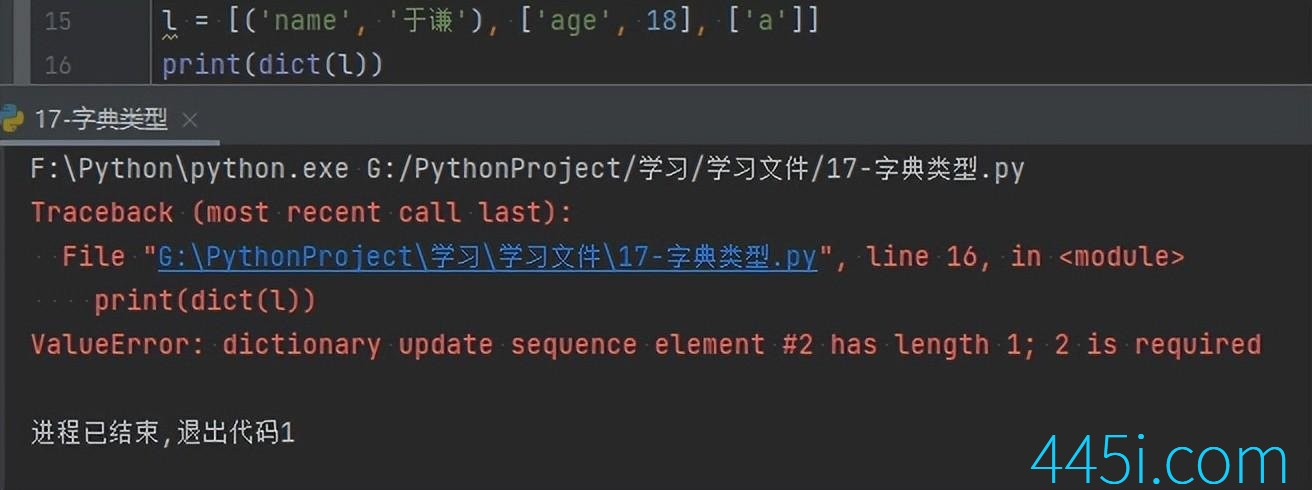``````dic = {'a': 1, 'b': 2}
dic['c'] = 3
print(dic)``````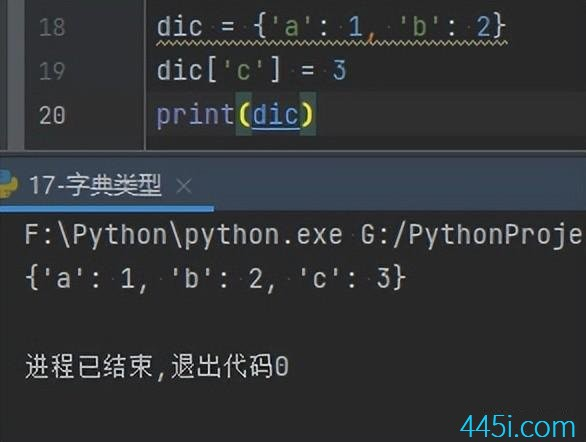``````dic = {'a': 1, 'b': 2}
print(dic['a'])````````````dic = {'a': 1, 'b': 2, 'c': 3}
print(dic.get('a'))``````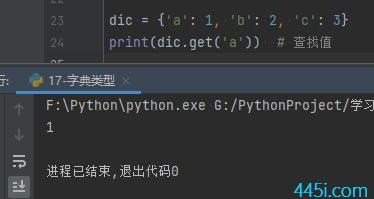``````dic = {'a': 1, 'b': 2,'b':3}
print(dic)``````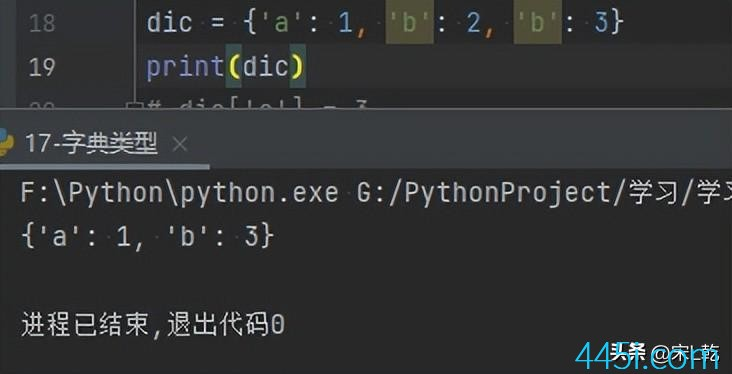### 觉得文章有用就打赏一下文章作者

#### 支付宝扫一扫打赏#### 微信扫一扫打赏